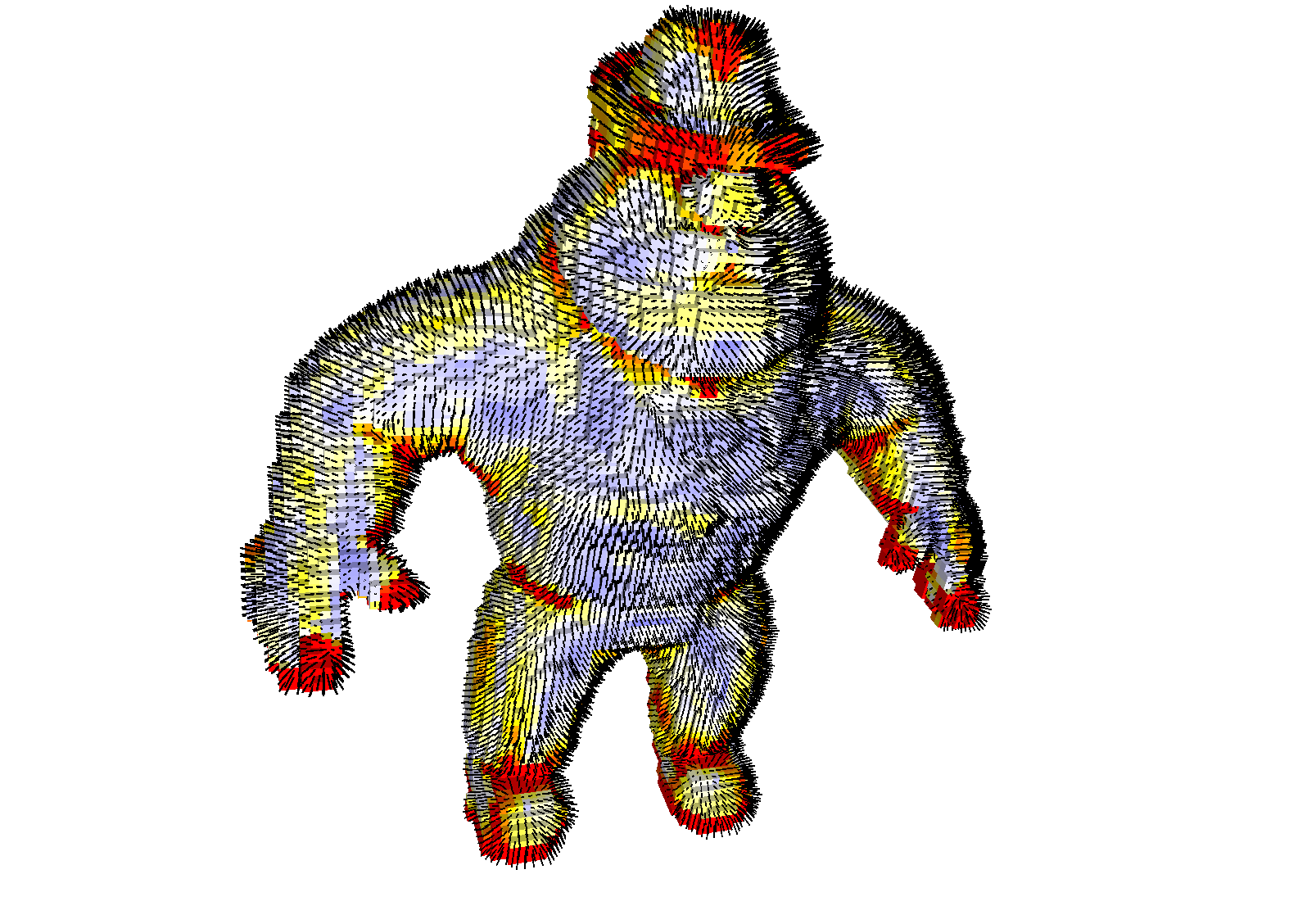DGtal  1.2.beta
geometry/surfaces/dvcm-3d.cpp

This example shows the computation of the VCM of a digital surface read from a .vol file. The normal is estimated from the diagonalization of the VCM tensor, while the orientation is deduced from the orientation of the trivial surfel normals. Feature detection is achieved with the eigenvalues of the VCM. A red color indicates a feature. Normals are displayed as black lines.

See also
Voronoi Covariance Measure of a digital surface
\$ ./examples/geometry/surfaces/dvcm-3dNormal vector and feature detection with Voronoi Covariance Measure.
#include <iostream>
#include "DGtal/base/Common.h"
#include "DGtal/helpers/StdDefs.h"
#include "DGtal/kernel/BasicPointPredicates.h"
#include "DGtal/math/linalg/EigenDecomposition.h"
#include "DGtal/topology/helpers/Surfaces.h"
#include "DGtal/topology/DigitalSurface.h"
#include "DGtal/topology/ImplicitDigitalSurface.h"
#include "DGtal/images/ImageSelector.h"
#include "DGtal/images/IntervalForegroundPredicate.h"
#include "DGtal/geometry/volumes/distance/ExactPredicateLpSeparableMetric.h"
#include "DGtal/geometry/surfaces/estimation/VoronoiCovarianceMeasureOnDigitalSurface.h"
#include "DGtal/io/colormaps/GradientColorMap.h"
#include "DGtal/io/viewers/Viewer3D.h"
#include "DGtal/io/readers/GenericReader.h"
#include "ConfigExamples.h"
using namespace std;
using namespace DGtal;
int main( int argc, char** argv )
{
QApplication application(argc,argv);
typedef Z3i::Space Space;
typedef Z3i::Point Point;
typedef KSpace::Cell Cell;
typedef ImplicitDigitalSurface< KSpace, ThresholdedImage > DigitalSurfaceContainer;
typedef ExactPredicateLpSeparableMetric<Space, 2> Metric; // L2-metric type
typedef functors::HatPointFunction<Point,double> KernelFunction; // chi function type
typedef VoronoiCovarianceMeasureOnDigitalSurface< DigitalSurfaceContainer, Metric,
KernelFunction > VCMOnSurface;
typedef VCMOnSurface::Surfel2Normals::const_iterator S2NConstIterator;
string inputFilename = examplesPath + "samples/Al.100.vol";
trace.info() << "File = " << inputFilename << std::endl;
int thresholdMin = 0;
trace.info() << "Min image thres. = " << thresholdMin << std::endl;
int thresholdMax = 1;
trace.info() << "Max image thres. = " << thresholdMax << std::endl;
const double R = 20;
trace.info() << "Big radius R = " << R << std::endl;
const double r = 3;
trace.info() << "Small radius r = " << r << std::endl;
const double trivial_r = 3;
trace.info() << "Trivial radius t = " << trivial_r << std::endl; // for orienting the directions given by the tensor.
const double T = 0.1;
trace.info() << "Feature thres. T = " << T << std::endl; // threshold for displaying features as red.
const double size = 1.0; // size of displayed normals.
KSpace ks;
// Reads the volume
trace.beginBlock( "Loading image into memory and build digital surface." );
Image image = GenericReader<Image>::import(inputFilename );
ThresholdedImage thresholdedImage( image, thresholdMin, thresholdMax );
trace.beginBlock( "Extracting boundary by scanning the space. " );
ks.init( image.domain().lowerBound(),
image.domain().upperBound(), true );
SurfelAdjacency<KSpace::dimension> surfAdj( true ); // interior in all directions.
Surfel bel = Surfaces<KSpace>::findABel( ks, thresholdedImage, 10000 );
DigitalSurfaceContainer* container =
new DigitalSurfaceContainer( ks, thresholdedImage, surfAdj, bel, false );
DigitalSurface< DigitalSurfaceContainer > surface( container ); //acquired
trace.info() << "Digital surface has " << surface.size() << " surfels." << std::endl;
Surfel2PointEmbedding embType = Pointels; // Could be Pointels|InnerSpel|OuterSpel;
Metric l2; // Euclidean L2 metric
KernelFunction chi( 1.0, r ); // hat function with support of radius r
VCMOnSurface vcm_surface( surface, embType, R, r,
chi, trivial_r, l2, true /* verbose */ );
trace.beginBlock( "Displaying VCM" );
Viewer3D<> viewer( ks );
Cell dummy;
viewer.setWindowTitle("3D VCM viewer");
viewer << SetMode3D( dummy.className(), "Basic" );
viewer.show();
grad.addColor( Color( 128, 128, 255 ) );
grad.addColor( Color( 255, 255, 255 ) );
grad.addColor( Color( 255, 255, 0 ) );
grad.addColor( Color( 255, 0, 0 ) );
RealVector lambda; // eigenvalues of chi-vcm
for ( S2NConstIterator it = vcm_surface.mapSurfel2Normals().begin(),
itE = vcm_surface.mapSurfel2Normals().end(); it != itE; ++it )
{
Surfel s = it->first;
Point kp = ks.sKCoords( s );
RealPoint rp( 0.5 * (double) kp[ 0 ], 0.5 * (double) kp[ 1 ], 0.5 * (double) kp[ 2 ] );
RealVector n = it->second.vcmNormal;
vcm_surface.getChiVCMEigenvalues( lambda, s );
double ratio = lambda[ 1 ] / ( lambda[ 0 ] + lambda[ 1 ] + lambda[ 2 ] );
viewer.setFillColor( grad( ratio > T ? T : ratio ) );
viewer << ks.unsigns( s );
n *= size;
viewer.setLineColor( Color::Black );
viewer.addLine( rp + n, rp - n, 0.1 );
}
viewer << Viewer3D<>::updateDisplay;
application.exec();
return 0;
}
// //